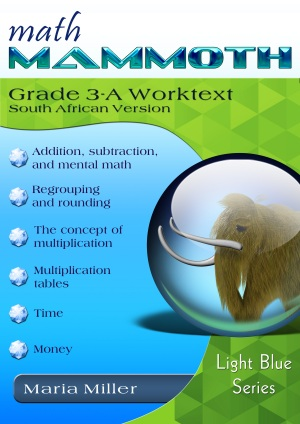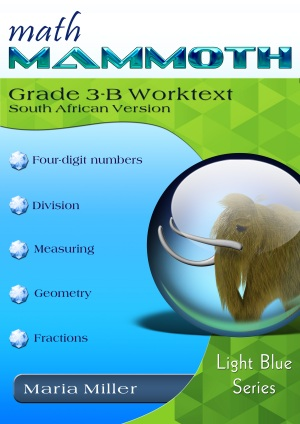^

You are here: HomeMM South African Version → Grade 3

# Math Mammoth Grade 3, South African Version

Math Mammoth Grade 3, South African Version is a complete maths program for 3rd grade. It consists of two student worktexts (A and B), a separate answer key for each worktext, tests, mixed revisions, and in the download version, an additional worksheet maker (Internet access required) to make extra practice worksheets when needed.182 pages
157 lesson pages164 pages
138 lesson pages

## Prices & ordering

(part A and B worktexts, answer keys, tests, cumulative revisions, a worksheet maker, and Soft-Pak)

Third grade is a time for learning and mastering two (mostly new) operations: multiplication and division within 100. The student also deepens his understanding of addition and subtraction, and uses those in many different contexts, such as with money, time, and geometry.

The main areas of study in Math Mammoth Grade 3 are:

1. Students develop an understanding of multiplication and division of whole numbers through problems involving equal-sized groups, arrays, and area models. They learn the relationship between multiplication and division, and solve many word problems involving multiplication and division (chapters 2, 3, and 9).
2. Students develop an understanding of fractions, beginning with unit fractions. They use visual fraction models and study fractions on a number line. Students also compare fractions by using visual fraction models and strategies based on noticing equal numerators or denominators (chapter 10).
3. Students learn the concepts of area and perimeter. They relate area to multiplication and to addition, recognise perimeter as a linear measure (in contrast with area), and solve problems involving area and perimeter (chapter 7).
4. Students fluently add and subtract within 1 000, both mentally and in columns (with regrouping). They learn to add and subtract 4-digit numbers, and use addition and subtraction in problem solving (chapters 1 and 6).

Additional topics we study are time (chapter 4), money (chapter 5), measuring (chapter 8), and bar graphs and picture graphs (in various chapters).

Please also see the table of contents for 3-A and 3-B (in the sample files), which will let you see the topics covered in more detail.

Some main features of Math Mammoth complete curriculum (Light Blue series) are:

• focuses on understanding of mathematical concepts
• uses clear explanations, lots of visual exercises and pattern exercises
• mastery oriented: concentrates at length on a topic, with very few topics per grade
• emphasizes mental maths and developing number sense
• very little teacher preparation needed

The downloadable version includes a worksheet maker (Internet access required) that lets you make extra practice worksheets for many of the topics in the curriculum.

The PDFs in the download version are enabled for annotation. This means that if you prefer, the student can fill them in on the computer, using the typewriter and drawing tools in Acrobat Reader version 9 or greater.

## South Africa

Math Mammoth South African version has been customized to South Africa in the following manners:

• The names used are South African names (such as Ansie and Jali).
• The currency used in word problems is rand. The money chapter teaches both rand and cents.
• The material is "all metric". In other words, the US customary measuring units are not used.
• Spelling is British English instead of American English.
• Paper size is A4.
• Geographic locations used emphasise South African locations (such as Pretoria, Johannesburg).

Please note that the curriculum is not following the South African official syllabus for 3rd grade maths. Instead, it simply is a copy of the US version of Math Mammoth Grade 3. For the most part, Math Mammoth exceeds South African standards. Some standards may not be covered.## Manipulatives

Here is a list of manipulatives that are needed or recommended for grade 3. Measuring tapes, scales, and measuring cups are only used in the measuring chapter.

1. A non-digital clock or other analog clock where you can turn the minute hand and the hour hand will turn accordingly (optional; for 3-A).
2. A ruler that measures in centimetres (for 3-B).
3. A measuring tape that measures in metres (for 3-B).
4. A kitchen scales that measures in grams (for 3-B).
5. A measuring cup that measures in millilitres (for 3-B).
6. Fraction manipulatives. The curriculum contains printables to make your own fraction manipulatives. The text uses visual pie models extensively, and students even learn to draw them themselves for the exercises, therefore making or buying the manipulatives is entirely optional (optional; for 3-B).

WAIT!

Receive my monthly collection of math tips & resources directly in your inbox — and get a FREE Math Mammoth book!You can unsubscribe at any time.

### Math Mammoth Tour

Confused about the different options? Take a virtual email tour around Math Mammoth! You'll receive:

An initial email to download your GIFT of over 400 free worksheets and sample pages from my books. Six other "TOURSTOP" emails that explain the important things and commonly asked questions concerning Math Mammoth curriculum. (Find out the differences between all these different-colored series!)

This way, you'll have time to digest the information over one or two weeks, plus an opportunity to ask me personally about the curriculum.
A monthly collection of math teaching tips & Math Mammoth updates (unsubscribe any time)### "Mini" Math Teaching Course

This is a little "virtual" 2-week course, where you will receive emails on important topics on teaching math, including:

- How to help a student who is behind
- Troubles with word problems
- Teaching multiplication tables
- Why fractions are so difficult
- The value of mistakes
- Should you use timed tests
- And more!

A GIFT of over 400 free worksheets and sample pages from my books right in the very beginning.A monthly collection of math teaching tips & Math Mammoth updates (unsubscribe any time)
Enter your email to receive math teaching tips, resources, Math Mammoth news & sales, humor, and more! I tend to send out these tips about once monthly, near the beginning of the month, but occasionally you may hear from me twice per month (and sometimes less often).• A GIFT of over 400 free worksheets and sample pages from my books.